Latest Banking jobs   »

# Reasoning Ability Quiz For SBI Clerk Prelims 2022- 9th May

Directions (1-5): In the following questions assuming the given statement to be true, find which of the conclusion(s) among given conclusions is/are definitely true and then give your answers accordingly.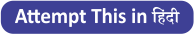Q1. Statements: E ≤ F = G, C ≥ D = E, G > H < I = J, A = B ≥ C
Conclusions:
I. A > E
II. A = E

(a) Only I is true
(b) Only II is true
(c) Either I or II is true
(d) Neither I nor II is true
(e) Both I and II are true

Q2. Statements: G = H < K, S > D ≥ F ≥ G, K ≤ L < P
Conclusions:
I. D > H
II. G < P

(a) Only I is true
(b) Only II is true
(c) Either I or II is true
(d) Neither I nor II is true
(e) Both I and II are true

Q3. Statements: Y > H = N, T ≤ G = B ≤ Y, N ≥ U ≤ J = M
Conclusions:
I. Y > U
II. G ≤ J

(a) Only I is true
(b) Only II is true
(c) Either I or II is true
(d) Neither I nor II is true
(e) Both I and II are true

Q4. Statements: P ≤ F ≤ G, D > N > G > O > T
Conclusions:
I. N > F
II. D > P

(a) Only I is true
(b) Only II is true
(c) Either I or II is true
(d) Neither I nor II is true
(e) Both I and II are true

Q5. Statements: D > H > V, W ≥ P ≥ H < R
Conclusions:
I. W < V
II. V < R

(a) Only I is true
(b) Only II is true
(c) Either I or II is true
(d) Neither I nor II is true
(e) Both I and II are true

Directions (6-10): In each question below, some statements are given followed by some conclusions. You have to take the given statements to be true even if they seem to be at variance with commonly known facts. Read all the conclusions and then decide which of the given conclusions logically follows/follow from the given statements, disregarding commonly known facts.

Q6. Statements: Some Match are Lost. No Lost is High. Only a few Rounds are Match.
Conclusions:
I. No Match is High.
II. All Round are High.

(a) If only conclusion I follows.
(b) If only conclusion II follows.
(c) If either conclusion I or II follows.
(d) If neither conclusion I nor II follows.
(e) If both conclusions I and II follow.

Q7. Statements: Some Match are Lost. No Lost is High. Only a few Rounds are Match.
Conclusions:
I. All Round is Lost.
II. All Lost are Match.
(a) If only conclusion I follows.
(b) If only conclusion II follows.
(c) If either conclusion I or II follows.
(d) If neither conclusion I nor II follows.
(e) If both conclusions I and II follow.

Q8. Statements: All Mats are Ground. No Mat is Inside. Only a few Grounds are Plains.
Conclusions:
I. Some Ground is not Inside.
II. Some Plains are Inside.
(a) If only conclusion I follows.
(b) If only conclusion II follows.
(c) If either conclusion I or II follows.
(d) If neither conclusion I nor II follows.
(e) If both conclusions I and II follow.

Q9. Statements: Some Blacks are Blue. Some Blue are Green. Some Green are Black.
Conclusions:
I. Some Black is Green.
II. Some Green is Blue.
(a) If only conclusion I follows.
(b) If only conclusion II follows.
(c) If either conclusion I or II follows.
(d) If neither conclusion I nor II follows.
(e) If both conclusions I and II follow.

Q10. Statements: Only a few Juices are Shakes. All Shakes are Drinks. All Shakes are Smoothies.
Conclusions:
I. Some Drinks are Juices.
II. Some Juices are Smoothies.
(a) If only conclusion I follows.
(b) If only conclusion II follows.
(c) If either conclusion I or II follows.
(d) If neither conclusion I nor II follows.
(e) If both conclusions I and II follow.

Directions (11-12): Study the following data carefully and answer the questions accordingly.
Point V is 8m west of point Y. Point M is 10m south of point Y. Point B is 12m west of point F and 5m south of point T. Point M is 10m east of point T.

Q11. Point Y is in which direction of point B?
(a) North-West
(b) South
(c) South-West
(d) North-East
(e) Can’t be determined

Q12. What is the shortest distance between points M and F?
(a) 29m
(b) 15m
(c) 7√8m
(d) √29m
(e) Can’t be determined

Directions (13-15): Read the following information carefully and answer the questions given below.
Point J is 10m east of Point I. Point C is 20m east of Point D. Point I is 10m north of Point H. Point E is 20m south of Point D. Point F is 10m west of Point E. Point G is 10m south of Point F. Point M is 30m north of point J. Point H is 30m east of Point G.

Q13. Which of the following points are in a straight line?
(a) G, H, J
(b) D, C, M
(c) I, E, D
(d) E, J, I
(e) None of these

Q14. What is the shortest distance between points C and J?
(a) 13√17m
(b) 25m
(c) 10√13m
(d) 20m
(e) None of these

Q15. Point M is in which direction of point F?
(a) East
(b) South-West
(c) North
(d) North- East
(e) None of these

Solutions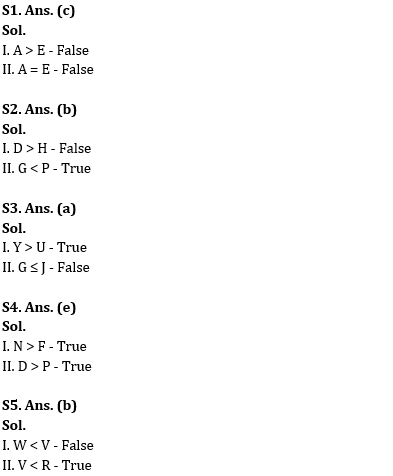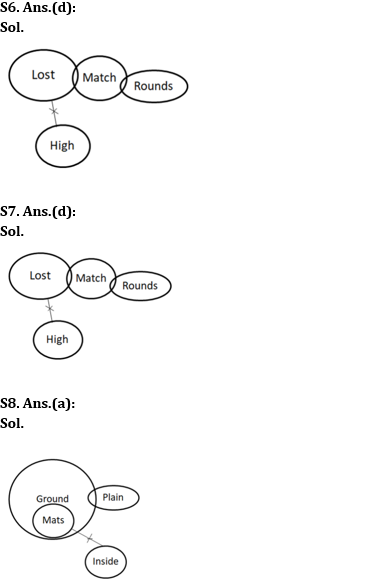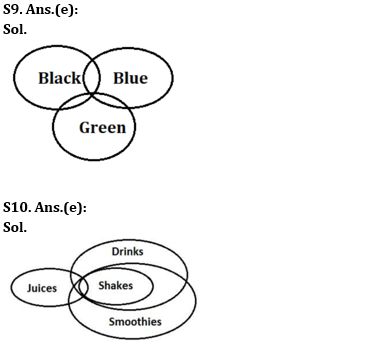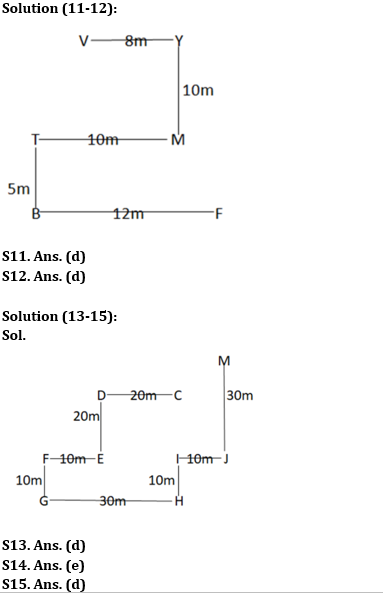#### Congratulations!

Incorrect details? Fill the form again here

•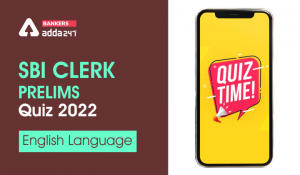English Quizzes For SBI Clerk Prelims 20...
•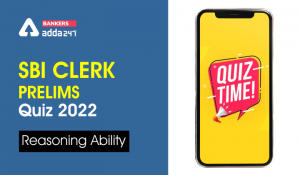Reasoning Ability Quiz For SBI Clerk Pre...
•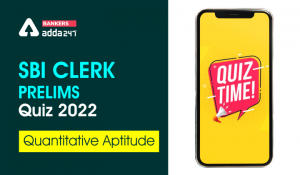Quantitative Aptitude Quiz For SBI Clerk...
•English Quizzes For SBI Clerk Prelims 20...
•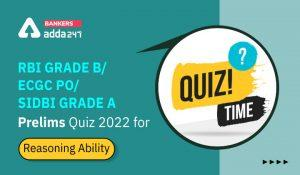Reasoning Ability Quiz For RBI Grade B/ ...
•Reasoning Ability Quiz For SBI Clerk Pre...